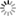##### Personal tools
You are here: Home Mathematical Programming

# Mathematical Programming

### - Overview

Mathematical programming, theoretical tool of management science and economics in which management operations are described by mathematical equations that can be manipulated for a variety of purposes. If the basic descriptions involved take the form of linear algebraic equations, the technique is described as linear programming. If more complex forms are required, the term nonlinear programming is applied.

The key difference between linear programming and nonlinear programming is that linear programming helps to find the best solution from a set of parameters or requirements with a linear relationship whereas nonlinear programming helps to find the best solution from a set of parameters with a linear relationship or request to find the best solution. nonlinear relationship.

Linear programming is an important concept in mathematical optimization techniques because it helps to find the optimal solution to a given problem. On the other hand, nonlinear programming is a mathematical method of finding an optimal solution by considering nonlinear constraints or objective functions.

Mathematical programming is used in planning production schedules, in transportation, in military logistics, and in calculating economic growth, by inserting assumed values for the variables in the equations and solving for the unknowns. Computers are widely used in obtaining solutions.

### - Linear Programming

Linear programming (LP, also called linear optimization) is a method to achieve the best outcome (such as maximum profit or lowest cost) in a mathematical model whose requirements are represented by linear relationships. Linear programming is a special case of mathematical programming (also known as mathematical optimization).

More formally, linear programming is a technique for the optimization of a linear objective function, subject to linear equality and linear inequality constraints. Its feasible region is a convex polytope, which is a set defined as the intersection of finitely many half spaces, each of which is defined by a linear inequality. Its objective function is a real-valued affine (linear) function defined on this polyhedron. A linear programming algorithm finds a point in the polytope where this function has the smallest (or largest) value if such a point exists.

### - Nonlinear Programming

[More to come ...]

##### Document Actions